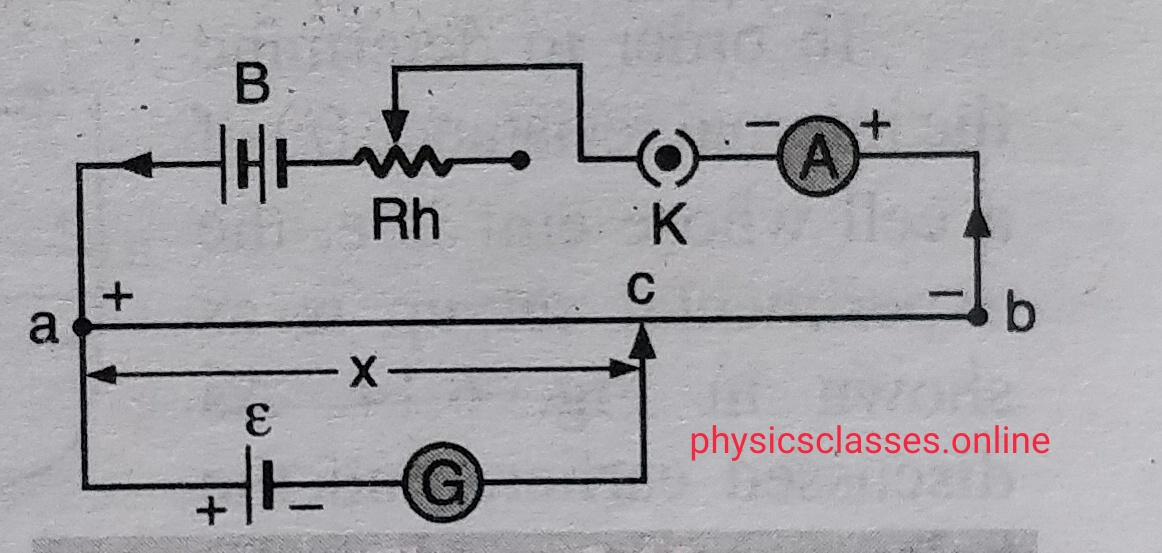Categories

## USES OF POTENTIOMETERPOTENTIOMETER USE-1

USES OF POTENTIOMETER Uses of potentiometer – Measurement of emf of cell-         Let , E is the  emf of  driver cell  and ,  E1  is the emf of cell to be measured Here potential gradient K= [ {E / (R+r)} r]/l . Let l1  is the length of the wire from […]